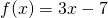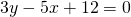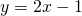# Unit 1 Review Solving Equations

Being able to discuss numbers hypothetically – using a letter to represent a number – underpins much of this course.

Activity: Algebra Review Solving equations

Use this applet to find the kinds of equations that you need to practice most.

Use this one as a practice test.

It’s great to be able to solve equations for equations’ sake. However in the course we encountered many problems that could be solved by setting up and solving an equation. Here are some examples. Each example also require that you understand some other area of knowledge –  what an arithmetic sequence is; or what is meant by; or how trigonometry works; or understand the term ‘perimeter’, etc.

# Solving Equations in Context

Printable:  Solving Equations in Context Solutions: Solving Eq in Context Solution

1. This right angled triangle has one side length 9 cm, and one angle 18°.From trigonometry, we know that. Solve this equation to find the length of the side markedcm.

2. This right angled triangle has one side marked 9 cm, and an angle 24°.From trigonometry, we know that. Solve this equation to find the length of the side markedcm.

3. An arithmetic sequence has general term. The number 61 is part of this sequence. What term is the number 61? Set up an equation and solve it.

4. An arithmetic sequence has general term. The number 31 is a term in this sequence. What term is the number 31? Set up an equation and solve it.

5. The arithmetic sequencesandhave a common term – that is, for some value of,. Set up an equation to find the value of, then find the value of the term.

6. The arithmetic sequencesanddo not share a common term. How can this be proved?

7. The rectangle and the square shown below have the same perimeter. The sides of the rectangle and the side length of the square are given in terms of an unknown number. Set up an equation to find the value of. Then find the dimensions of each shape, and the perimeter.8. A rectangle has perimeter 27.6 cm. The length is 5 times as long as the width. Set up an equation with this information and solve it to find the length and width of the rectangle.9. A function is defined as. Findwhen.

10. A function is defined as. Find the value ofwhen.

11. A straight line has equation. Set up an equation to calculate theintercept of the line.

12. A straight line has equation. Set up an equation to calculate theintercept.

13. A straight line has equation. Calculate theintercept and theintercept.

14. The straight linesandhave a point in common – a point where the lines intersect each other. Set up an equation to find thevalue of this point. Calculate thevalue.

15. The straight linesandhave a point in common. Set up an equation to find thevalue of this point. Calculate thevalue.